# Center of mass of System of particles

## (1) Introduction to center of mass

• Until now we have focused on describing motion of a single particle in one, two or three dimensions. By particle we mean to say that it has a size negligible in comparison to the path travelled by it.
• When we studied Law's of motion we have applied them even to the bodies having finite size imagining that motion of such bodies can be described in terms of motion of particles.
• While doing so we have ignored the the internal structure of such bodies. Any real body we encounter in our daily life has a finite size and idealized model of particle is inadequate when we deal with motion of real bodies of finite size .
• Real bodies of finite size can also be regarded as the system of particles. While studying system of particles we will not concentrate on each and every particle of the system instead we will consider the motion of system as a whole.
• Large number of problems involving extended bodies or real bodies of finite size can be solved by considering them as Rigid Bodies. We define rigid body as a body having definite and unchanging shape.
• A rigid body is a rigid assembly of particles with fixed inter-particle distances. In actual bodies deformations do occur but we neglect them for the sake of simplicity.
• In this chapter we will study about center of mass of system of particles, motion of center of mass and about collisions.

### Introductory concepts

Let us learn about few important definitions before moving further

1. Particle :- A particle is defined as an object whose mass is finite and it has a size that is negligible in comparison to the path travelled by it.
2. System:- A system is a collection of a very large number of particles which mutually interact with one another. So, a body of finite size can be regarded as a system because it is made up of large number of particles interacting with one another.
3. Internal forces:- The mutual forces exerted by the particles of system on one another are called internal forces.
4. External forces:- The outside forces exerted on an object by any external agency is called external forces. Such a force changes the velocity of an object.

## (2) Center of mass definition

• Consider a body consisting of large number of particles whose mass is equal to the total mass of all the particles. When such a body undergoes a translational motion the displacement is produced in each and every particle of the body with respect to their original position.
• If this body is executing motion under the effect of some external forces acting on it then it has been found that there is a point in the system , where whole mass of the system is supposed to be concentrated. The nature the motion executed by the system remains unaltered when force acting on the system is directly applied to this point. Such a point of the system is called center of mass of the system.
• Hence for any system Center of mass is the point where whole mass of the system can be supposed to be concentrated and motion of the system can be defined in terms of the center of mass.

### Difference between center of mass and center of gravity

The center of mass of a body i a point where whole mass of a body may be assumed to be concentrated for describing its translational motion. On the other hand the center of gravity is the point at which the resultant of the gravitational forces on all the particles of the body acts.
Please note that for many objects, these two points are in exactly the same place. But they're only the same when the gravitational field is uniform across an object. For example in uniform gravitational field such as that of the earth on a small body , the center of gravity coincides with the center of mass.

### (3) Center of mass Formula

#### (i) Position of center of mass for two particle system

consider a system of two point masses (or particles) $m_1$ and $m_2$ , whose position vectors at time $t$ with reference to the origin $O$ of the inertial frame are $\vec{r_1}$ and $\vec{r_2}$ respectively as shown below in the figure.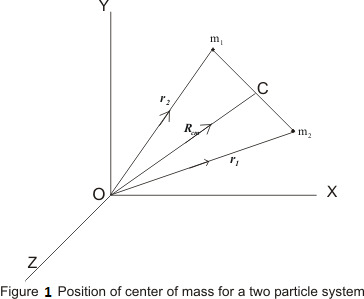The total force $(\vec{F_1})_{tot}$ that is acting on point mass $m_1$ consists of two parts
(i) A force $(\vec{F_1})_{ext}$ which appears because of some agency acting on the system
(ii) A force $\vec{F_{12}}$ which appears because of point charge $m_2$. This force is internal force of the two particle system.
So, Total force is
\begin{equation} (\vec{F_1})_{tot}=\vec{F_{12}}+(\vec{F_1})_{ext} \tag{1} \end{equation} Similarly for point mass $m_2$ \begin{equation} (\vec{F_2})_{tot}=\vec{F_{21}}+(\vec{F_2})_{ext} \tag{2} \end{equation} Now we will write the equation of motion for point mass $m_1$ using newton's second law of motion
\begin{equation} \frac{d}{dt}(m_1\vec{v_1})=\vec{F_1})_{tot} \tag{3} \end{equation} Similarly for point mass $m_2$ \begin{equation} \frac{d}{dt}(m_2\vec{v_2})=\vec{F_2})_{tot} \tag{4} \end{equation} Adding equations (3) and (4) we get
\begin{equation} \frac{d}{dt}(m_1\vec{v_1})+\frac{d}{dt}(m_2\vec{v_2}) = \vec{F_1})_{tot} +\vec{F_2})_{tot} \\ \frac{d}{dt}(m_1\vec{v_1} + m_2\vec{v_2}) = \vec{F_1})_{tot} +\vec{F_2})_{tot} \end{equation} From equation (1) and (2) \begin{equation} \frac{d}{dt}(m_1\vec{v_1} + m_2\vec{v_2}) = \vec{F_{12}}+(\vec{F_1})_{ext} + \vec{F_{21}}+(\vec{F_2})_{ext} \end{equation} But from Newton's third law of motion we know that $\vec{F_{21}}=-\vec{F_{12}}$ so now we have
\begin{equation} \frac{d}{dt}(m_1\vec{v_1} + m_2\vec{v_2}) = (\vec{F_1})_{ext} + (\vec{F_2})_{ext} \\ \end{equation} Or,
\begin{equation} \frac{d}{dt}(m_1\vec{v_1} + m_2\vec{v_2}) = \vec{F} \tag{5} \end{equation} where, $\vec{F}=(\vec{F_1})_{ext} + (\vec{F_2})_{ext}$ is the total external force.
You must remember that $(\vec{F_1})_{ext}$ and $(\vec{F_2})_{ext}$ are acting on different points of the system and we are adding them as free vectors.
Now the velocity vectors are given by \begin{equation} \vec{v_1}=\frac{d\vec{r_1}}{dt} \quad \text{and} \quad \vec{v_2}=\frac{d\vec{r_2}}{dt} \end{equation} So, we have
\begin{align} m_1\vec{v_1} + m_2\vec{v_2} &= m_1\frac{d\vec{r_1}}{dt} + m_2\frac{d\vec{r_2}}{dt} \\ &=\frac{d}{dt}(m_1\vec{r_1})+\frac{d}{dt}(m_2\vec{r_2}) \\ &= \frac{d}{dt}(m_1\vec{r_1} + m_2\vec{r_2}) \end{align} From equation (5) we have \begin{equation} \vec{F}=\frac{d^2}{dt^2}(m_1\vec{r_1} + m_2\vec{r_2}) \end{equation} or,
\begin{equation} \vec{F}=M\frac{d^2}{dt^2}\frac{(m_1\vec{r_1} + m_2\vec{r_2})}{M} \end{equation} This equation is clearly the equation of motion of a hypothetical object of mass $M=m_1+m_2$. The position of this point at any time is given by position vector $\vec{R_{CM}}$ such that
\begin{equation} \vec{R_{CM}}=\frac{(m_1\vec{r_1} + m_2\vec{r_2})}{M} \tag{6} \end{equation} the point whose position is defined by $\vec{R_{CM}}$ is called the center of mass of two particle system. So, equation 6 gives the center of mass formula for a two particle system at any time $t$. It is a point at which the total external force is supposed to be acting.

Note:- It should be noted that it is not necessary that there may be a material particle at the center of mass of the system. Center of mass of a system is a hypothetical point and it may lie inside the system or outside it.

### Center of mass formula observation

1. The position vector $\vec{R_{CM}}$ of the center of mass $C$ of two particles is given by
$$\vec{R_{CM}}=\frac{m_1\vec{r_1}+m_2\vec{r_2}}{m_1+m_2}$$ From above equation we can see that the position vector of a system of particles is the weighted average of the position vectors of the particles of which the system is made up of. Each particle in the system makes contribution proportional to its mass.
2. From above equation we have
$$(m_1+m_2)\vec{R_{CM}}=m_1\vec{r_1}+m_2\vec{r_2}$$ Here we can see that the product of the total mass of the system and the position vector of its center of mass is equal to the sum of the products of individual masses and their respective position vectors.
3. Again if $m_1=m_2=m$ , then
$$\vec{R_{CM}}=\frac{\vec{r_1}+\vec{r_2}}{2}$$ Thus , center of mass of two equal masses lie exactly at the center of the line joining the two masses.
4. If $(x_1,y_1)$ and $(x_2,y_2)$ are the coordinates of the locations of two particles , then coordinates of their center of mass is given by
$$x_{CM}=\frac{m_1x_1 + m_2x_2}{m_1 +x_1}$$ $$y_{CM}=\frac{m_1y_1 + m_2y_2}{m_1 +x_1}$$

#### (ii)Position of center of mass for many particle system

• Consider a many particle system made up of number of particles as shown below in the figure. Let m1 , m2 , m3 , . . . . . . . . . . . . . , mn be the masses of the particles of system and their respective position vectors w.r.t. origin are r1 , r2 , r3 , . . . . . . . . . . . . . . . . . , rn.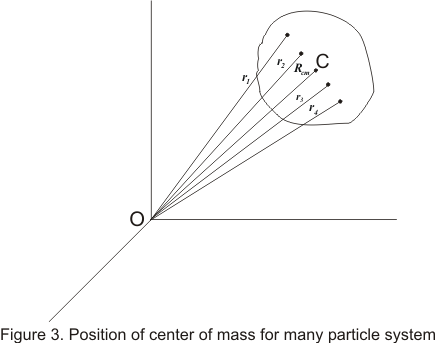• Because of the definition of center of mass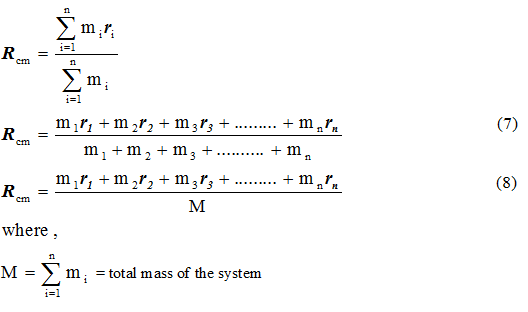### Some conceptual questions on center of mass

Question 1 Is center of mass a hypothetical point or it really exists?
Answer The concept of center of mass of a system enable us to discuss overall motion of the system. It is defined as a point at which the entire mass of the bodies is supposed to be concentrated. So it is a hypothetical point only. So it is not necessary that the total mass of the system is to be exactly present at the center of mass. Hence we can say that it is a hypothetical point.
Question 2 How is the center of mass depends on the relative distance between the particles?
Answer Consider we have to find out the center of mass of the two masses having mass m Kg and 5m Kg. Now center of mass will lie closer to the heavier body (5m Kg) because center of mass is a point about which the whole mass of the system is balanced.
Question 3 Write two factors on which center of mass of a body does not depend.
Question 4 Give the location of a center of mass of a ring? does it it lie inside the body?
Answer The center of mass of the ring lies in the center of the circle outlined by the ring. It is at the middle of the ring and does not lie inside the body. This is because, center of mass is a mathematical point where the mass of a body seems to be concentrated at.
Question 5 Define motion of center of mass of a fire cracker that explode in air before and after exposition"?
Answer A fire cracker that explodes in air, in the absence of air drag , the center of mass of the fragments would continue to follow the original parabolic path, until the fragmented parts began to touch the ground.

## Important points about center of mass.

1. The position of center of mass is independent of the co-ordinate system you choose.
2. The position of center of mass depends upon the shape of the body and distribution of mass across the body. For example: The center of mass of a circular disc is inside the material of the body while that of a circular ring lies outside the material of the body.
3. Symmetrical bodies in which the distribution of mass is homogeneous, the center of mass coincides with the geometrical center or center of symmetry of the body.
4. Center of mass of a body changes its position only under translatory motion but not under rotatory motion because here the body is rotating in its position and not moving (changing its co-ordinates) so its Center of mass remains at the same position.
5. Position of center of mass for different symmetrical bodies
 S.No. Body Position of center of mass 1 Uniform hollow sphere Center of sphere 2 Uniform solid sphere Center of sphere 3 Uniform circular ring Center of ring 4 Uniform circular disc Center of disc 5 Uniform rod Center of rod 6 A plane lamina Point of inter section of diagonals 7 Triangular plane lamina Point of inter section of medians 8 Rectangular or cubical block Points of inter section of diagonals 9 Hollow cylinder Middle point of the axis of cylinder 10 Solid cylinder Middle point of the axis of cylinder

## Center of mass solved problems

Question 1 Find the center of mass of a uniform semi-circular ring of radius R and mass M.

Solution: Let us consider the center of the ring as origin.

We would have to solve this problem using calculus method. For this consider a differential element of length $dl$ of the ring whose radius vector makes an angle $\theta$ with the x-axis. If the angle made by the length $dl$ is $d\theta$ at the center as shown below in the figure then,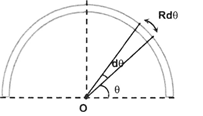$dl = Rd\theta$

Let $\lambda$ be the mass per unit length.

Then, mass of this element is $dm=\lambda Rd\theta$

Now center of mass of continuous system is given by the relation

\begin{align} {{X}_{CM}} &=\frac{1}{m}\int{xdm} \\ & =\frac{1}{m}\int\limits_{0}^{\pi }{(R\cos \theta )R\lambda d\theta }=0 \\ & {{Y}_{CM}}=\frac{1}{m}\int\limits_{0}^{\pi }{(R\sin \theta )\lambda Rd\theta } \\ & =\frac{\lambda {{R}^{2}}}{m}\int\limits_{0}^{\pi }{(sin\theta )d\theta }=\frac{\lambda {{R}^{2}}}{\lambda \pi R}[-\cos \theta ]_{0}^{\pi } \\ & \Rightarrow {{Y}_{CM}}=\frac{2R}{\pi } \\ \end{align}

Points to Note:- $m=\lambda \pi R$ as maximum angle that can be subtended by the ring is $\pi$ as you can note that we are taking limits of integration from $0$ to $\pi$.

Question 2 If the linear mass density of a rod of length L varies as $\lambda = A + Bx$ (where A and B are constants), then find the coordinates of center of mass.

Solution Let us first analyze the problem. Here we are given linear mass density and it is only a function of $x$. So we can safely conclude that

$Y_{CM}=0$ and $Z_{CM}=0$

So we have to find the center of mass for X-Coordinate. For this let us consider the figure given below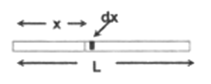To calculate center of mass for a continuous system of particles let us consider infinitesimal small element of length $dx$ and mass $dm$ and then use the center of mass equation below to calculate the center of mass for X-Coordinate.

\begin{align} & {{X}_{CM}}=\frac{\int\limits_{0}^{L}{xdm}}{\int\limits_{0}^{L}{dm}} \\ & dm=\lambda dx=(A+Bx)dx \\ & {{X}_{CM}}=\frac{\int\limits_{0}^{L}{x(A+Bx)dx}}{\int\limits_{0}^{L}{(A+Bx)dx}}=\frac{\frac{A{{L}^{2}}}{2}+\frac{B{{L}^{3}}}{3}}{AL+\frac{B{{L}^{2}}}{2}} \\ & {{X}_{CM}}=\frac{L(3A+2BL)}{3(2A+BL)} \\ \end{align}

Hence , co-ordinates of center of mass are $\left( \frac{L(3A+2BL)}{3(2A+BL)},0,0 \right)$ .

Question 3. If a man of mass m jumps to the ground from height h and his center of mass moves a distance d in the time taken by him to hit the ground show that the average force acting on him is mgh/d (assuming his retardation to be constant during his impact with the ground) .

Solution. Force is considered the change in potential energy, U, over a change in position, x. In other words it is the derivative of potential energy with respect to time.
F = dU/dx

here,U=mgh

change in position = change in position of center of mass = d

so average $force=\frac{mgh}{d}$

Question 4. A child is standing at one end of a long trolley moving with a speed v on a smooth horizontal track. If the child starts running towards the other end of the trolley with a speed u, the centre of mass of the system (trolley + child) will move with what speed?

Solution. When the child is standing on the trolley, both child and trolley are moving with the same velocity = v

So, their center of mass is also moving with the velocity of the vehicle,

$v_{cm}=\frac{mv+Mv}{m+M}=v$

the velocity of center of mass changes only if the system is subjected to net external force. But since the only forces involved in the child + trolley system are internal (action-reaction pair) when the child runs on the trolley. Therefore, the velocity of their centre of mass will remain unchanged. It will be v

## (4) center of mass equation (Position vector of center of mass in terms of co-ordinate components)

• Let in a system of many particles the co-ordinates of center of mass of the system be (Xcm,Ycm,Zcm) then position vector of centre of mass would be
Rcm=Xcmi+Ycmj+Zcmk                    (9)
and position vectors of various particles would be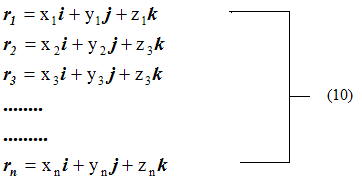• Putting the values from equation 9 and 10 in equation 6, 7 and 8 we get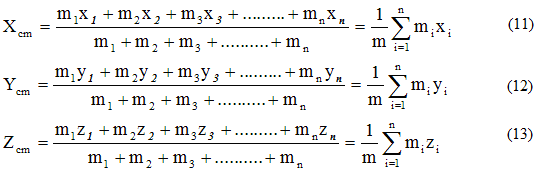• If in any system there are infinite particles of point mass and are distributed continuously also if the distance between them is infinitesimally small then summation in equations 6, 11, 12 and 13 can be replaced by integration. If r is the position vector of very small particle of mass dm of the system then position vector of centre of the system would be
and the value of its co-ordinates would be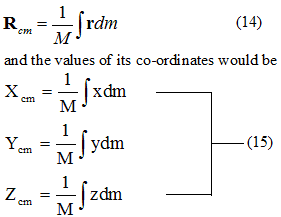• If ρ is the density of the system then dm=ρdV where dV is the very small volume element of the system then,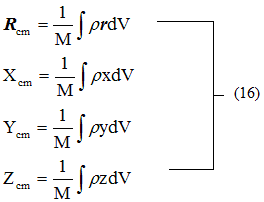• The center of mass of a homogeneous body (body having uniform distribution of mass) must coincide with the geometrical center of the body. In other words we can say that if the homogeneous body has a point , a line or plane of symmetry , then its centre of mass must lie at this point , line or plane of symmetry.
• The center of mass of irregular bodies and shape can be found using equations 14, 15, 16.

Some other resources you can look for reference are